Výsledky výzkumu a další informace nejenz oblasti přístupových telekomunikačních sítí.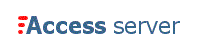ISSN 1214-9675
Server vznikl za podpory Grantové agentury ČR.
20. ročník
 Dnešní datum: 27. 06. 2022 Hlavní stránka | Seznam rubrik | Ke stažení | Odkazy

Doporučujeme
Knihu o FTTx

Matlab server - on-line výpočty a simulace

E-learning - on-line kurzy

Kontakt
KTT FEL ČVUT
Napište nám

Redakční rada - pokyny pro autory a recenzenty#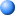Basic principles of fuzzy logic

Vydáno dne 01. 08. 2012 (56615 přečtení)

Fuzzy logic is a complex mathematical method that allows solving difficult simulated problems with many inputs and output variables. Fuzzy logic is able to give results in the form of recommendation for a specific interval of output state, so it is essential that this mathematical method is strictly distinguished from the more familiar logics, such as Boolean algebra. This paper contains a basic overview of the principles of fuzzy logic.

### Fuzzy Logic System

Today control systems are usually described by mathematical models that follow the laws of physics, stochastic models or models which have emerged from mathematical logic. A general difficulty of such constructed model is how to move from a given problem to a proper mathematical model. Undoubtedly, today’s advanced computer technology makes it possible; however managing such systems is still too complex.

These complex systems can be simplified by employing a tolerance margin for a reasonable amount of imprecision, vagueness and uncertainty during the modelling phase. As an outcome, not completely perfect system comes to existence; nevertheless in most of the cases it is capable of solving the problem in appropriate way. Even missing input information has already turned out to be satisfactory in knowledge-based systems.

Fuzzy logic allows to lower complexity by allowing the use of imperfect information in sensible way. It can be implemented in hardware, software, or a combination of both. In other words, fuzzy logic approach to problems’ control mimics how a person would make decisions, only much faster.

The fuzzy logic analysis and control methods shown in Figure 1 can be described as:

1. Receiving one or large number of measurements or other assessment of conditions existing in some system that will be analysed or controlled.
2. Processing all received inputs according to human based, fuzzy ”if-then” rules, which can be expressed in simple language words, and combined with traditional non-fuzzy processing.
3. Averaging and weighting the results from all the individual rules into one single output decision or signal which decides what to do or tells a controlled system what to do. The result output signal is a precise defuzzified value.

The following is Fuzzy Logic Control/Analysis Method diagram.Figure 1. The fuzzy logic Control-Analysis method

In order to operate fuzzy logic needs to be represented by numbers or descriptions. For example, speed can be represented by value 5 m/s or by description “slow”. Term “slow” can have different meaning if used by different persons and must be interpreted with respect to the observed environment. Some values are easy to classify, while others can be difficult to determine because of human understanding of different situations. One can say “slow”, while other can say “not fast” when describing the same speed. These differences can be distinguished with help of so-called fuzzy sets.

Usually fuzzy logic control system is created from four major elements presented on Figure 2: fuzzification interface, fuzzy inference engine, fuzzy rule matrix and defuzzification interface. Each part along with basic fuzzy logic operations will be described in more detail below.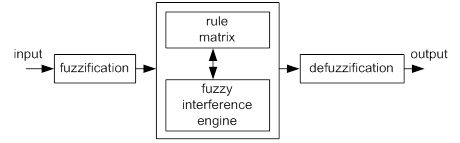Figure 2. Fuzzy logic controller 

### Fuzzy Logic Basic Operations

Below some basic information about fuzzy logic will be presented, while a comprehensive theory of fuzzy logic can be found in .

• Universe of Discourse

It is a range of all possible values considered as fuzzy system input.

• Fuzzy Set

A fuzzy set µ is a function from the reference set X to the unit interval, i.e.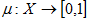(1)

µ(X) represents the set of all fuzzy sets of X.

• Membership Function

It is a graphical representation of fuzzy sets, µF(x).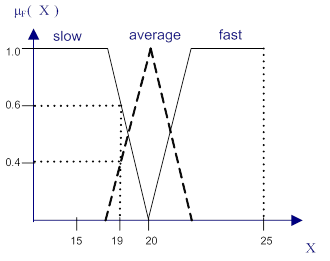Figure 3. An example of fuzzy logic membership function

Figure 3 shows the membership functions of three fuzzy sets, “slow”, “average”, and “fast”, for a fuzzy variable Velocity. The universe of discourse creates all possible values of Velocity, i.e., X = 19. For Velocity value 19 km/h, the fuzzy set “slow” has the membership value 0.6. Hence, µslow(19) = 0.6. Similarly, µaverage(19) = 0.4, and µfast(19) = 0.

• Support

The support of a fuzzy set F is the crisp set of all points in the Universe of Discourse U such that membership function of F does not equal zero(2)

• Crossover point

It is an element in U where its membership function equals 0.5.

• Centre

The centre of a fuzzy set F is the point (or points) at which µF(u) achieves its maximum value.

### Fuzzification Method

First phase of fuzzy logic proceeding is to deliver input parameters for given fuzzy system based on which the output result will be calculated. These parameters are fuzzified with use of pre-defined input membership functions, which can have different shapes. The most common are: triangular shape, however bell, trapezoidal, sinusoidal and exponential can be also used. Simpler functions will not require complex computing and will not overload the implementation. The degree of membership function is determined by placing a chosen input variable on the horizontal axis, while vertical axis shows quantification of grade of membership of the input variable. The only condition a membership function must meet is that it must vary between zero and one. The value zero means that input variable is not a member of the fuzzy set, while the value one means that input variable is fully a member of the fuzzy set.

With each input parameter there is a unique membership function associated. The membership functions associate a weighting factor with values of each input and the effective rules. These weighting factors determine the degree of influence or degree of membership (DOM) each active rule has. By computing the logical product of the membership weights for each active rule, a set of fuzzy output response magnitudes are produced. All that remains is to combine and defuzzify these output responses .

### Rule Matrix

The rule matrix is used to describe fuzzy sets and fuzzy operators in form of conditional statements. A single fuzzy if-then rule can be as follows

If x is A then y is Z,

where A is a set of conditions that have to be satisfied and Z is a set of consequences that can be inferred.

In rule with multiple parts, fuzzy operators are used to combine more than one input: AND = min, OR = max and NOT = additive complement. Geometrical demonstration of fuzzy operators is shown in Figure 4.Figure 4. Graphical interpretation of fuzzy operators

The rule matrix is a simple graphical tool for mapping the fuzzy logic control system rules. It accommodates two or more input variables and expresses their logical product (AND or OR) as one output response variable. The degree of membership for rule matrix output can take value of maximum, minimum of the degree of previous of the rule . It is often probable, that after evaluation of all the rules applicable to the input, we get more than one value for the degree of membership. In this case, the simulation has to take into consideration, all three possibilities, the minimum, the maximum or an average of the membership-degrees.

Inference Mechanisms

Inference mechanism allows mapping given input to an output using fuzzy logic. It uses all pieces described in previous sections: membership functions, logical operations and if-then rules. The most common types of inference systems are Mamdani and Sugeno. They vary in ways of determining outputs.

Mamdani method

Below examples are based on two fuzzy control rules in the form of

R1: if x is A1 and y is B1 then z is C1
R2: if x is A2 and y is B2 then z is C2

Result: z is C, where x equals x0 and y equals y0.

The firing levels of the rules, denoted by αi, i = 1, 2 are calculated by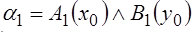(3)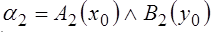(4)

The individual rule outputs are derived by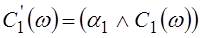(5)(6)

Then the overall system output is calculated by oring the individual rule outputs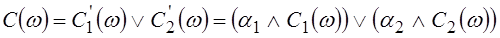(7)

Finally, to obtain a deterministic control action, chosen defuzziﬁcation mechanism must be implemented.

Sugeno method

Below examples are based on two fuzzy control rules in the form of

R1: if x is A1 and y is B1 then z is z1 = a1x1+b1y1
R2: if x is A2 and y is B2 then z is z2 = a2x2+b2y2

Result: z0, where x equals x0 and y equals y0.

The firing levels of the rule are calculated in the same way as in Mamdani method, based on (3) and (4) equations. The individual rule outputs are calculated from the below relationships(8)(9)

If there is n rules in the rule matrix, the crisp control result derived from the following equations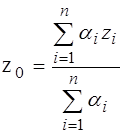(10)

where αi is a firing level of the i rule, and i = 1, …., n.

The Sugeno method works well with linear techniques, as it is computationally efficient. It is suitable to apply optimisation and adaptive techniques. Furthermore, it guarantees continuity of the output surface and it is well suited to mathematical analysis. An advantage of the Mamdani method is that is it intuitive and has widespread of acceptance. It can be well suited to human input .

### Defuzzification Mechanisms

Defuzzification task is to find one single crisp value that summarises the fuzzy set. There are several mathematical techniques available: centroid, bisector, mean, maximum, maximum and weighted average. Figure 5 demonstrate illustration of how values for each method are chosen.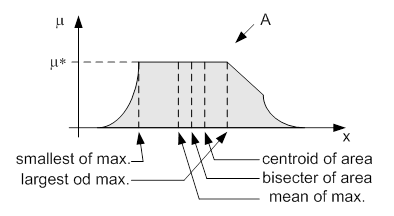Figure 5. Graphical demonstration of defuzzification methods 

Centroid defuzzification is the most commonly used method, as it is very accurate. It provides centre of the area under the curve of membership function. For complex membership functions it puts high demands on computation. It can be expressed by the following formula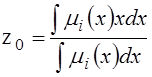(11)

where z0 is defuzzified output, ui is a membership function and x is output variable.

Bisector defuzzification uses vertical line that divides area under the curve into two equal areas.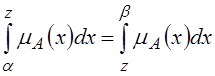(12)

Mean of maximum defuzzification method uses the average value of the aggregated membership function outputs.(13)

where x’ = {x; µA(x) = µ*}.

Smallest of maximum defuzzification method uses the minimum value of the aggregated membership function outputs.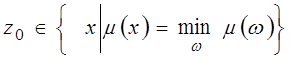(14)

Largest of maximum defuzzification method uses the maximum value of the aggregated membership function outputs.(15)

Weighted average defuzzification method, based on peak value of each fuzzy sets, calculates weighted sum of these peak values . According to these weight values and the degree of membership for fuzzy output, the crisp value of output is determined by the following formula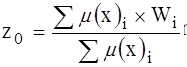(16)

where µi is the degree of membership in output singleton i, Wi and is the fuzzy output weight value for the output singleton i..

### Conclusion

Fuzzy Logic provides a completely different approach. One can concentrate on solving the problem rather than trying to model the system mathematically, if that is even possible. This almost invariably leads to quicker, cheaper solutions. Once understood, this technology is not difficult to implement and the results are usually quite surprising and more than satisfactory.

### References

 Fullér R., HASSANEIN H., ALI A.N.,: Neural fuzzy systems: towards IMT-advanced networks. Åbo: Åbo akademi, 1996, xxvii, 275 p. ISBN 95-165-0624-0.
 Wang L-X., HASSANEIN H., ALI A.N.,: Adaptive fuzzy systems and control: design and stability analysis. Englewood Cliffs, N.J: Prentice Hall, 1994, xxvii, 275 p. ISBN 978-013-1471-092.
 Ross T.J., HASSANEIN H., ALI A.N.,: Fuzzy logic with engineering applications: design and stability analysis. 3rd ed. Chichester (Royaume Uni): Wiley, 2010, xxvii, 275 p. ISBN 978-047-0748-510.
 Kaehler S.D.,: Fuzzy Logic Tutorial. Seattle Robotics Society [online]. 1998. ed. [cit. 2012-08-22]. Avaible at: http://www.seattlerobotics.org/encoder/mar98/fuz/flindex.html

Autor:        E. Kozlowska
Pracoviště: České vysoké učení technické v Praze, FEL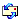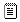Projekty a aktuality
01.03.2012: PROJEKT
Výzkum a vývoj nového komunikačního systému s vícekanálovým přístupem a mezivrstvovou spoluprací pro průmyslové aplikace TA02011015

01.01.2012: PROJEKT
Vývoj adaptabilních datových a procesních systémů pro vysokorychlostní, bezpečnou a spolehlivou komunikaci v extrémních podmínkách VG20122014095

09.10.2010: PROJEKT
Výzkum a vývoj datového modulu 10 Gbit/s pro optické a mikrovlnné bezdrátové spoje, FR-TI2/621

09.01.2010: PROJEKT
Sítě s femtobuňkami rozšířené o řízení interference a koordinaci informací pro bezproblémovou konektivitu, FP7-ICT-2009-4 248891

09.11.2008: PROJEKT
Ochrana člověka a techniky před vysokofrekvenčním zářením, FI-IM5/202

20.06.2008: Schválení
Radou pro výzkum a vývoj jako recenzovaný časopis

01.04.2007: PROJEKT
Pokročilá optimalizace návrhu komunikačních systémů pomocí neuronových sítí, GA102/07/1503

01.07.2006: Doplnění sekce pro registrované

12.04.2005: Zavedeno recenzování článků

30.03.2005: Výzkumný záměr
Výzkum perspektivních informačních a komunikačních technologií MSM6840770014

29.11.2004: Přiděleno ISSN

04.11.2004: Spuštění nové podoby Access serveru

18.10.2004: PROJEKT
Optimalizace přenosu dat rychlostí 10 Gbit/s, GA102/04/0773

04.09.2004: PROJEKT
Specifikace kvalitativních kritérií a optimalizace prostředků pro vysokorychlostní přístupové sítě, NPV 1ET300750402

04.06.2004: PROJEKT
Omezující faktory při širokopásmovém přenosu signálu po metalických párech a vzájemná koexistence s dalšími systémy, GA102/03/0434Tento web site byl vytvořen prostřednictvím phpRS - redakčního systému napsaného v PHP jazyce.
Na této stránce použité názvy programových produktů, firem apod. mohou být ochrannými známkami
nebo registrovanými ochrannými známkami příslušných vlastníků.